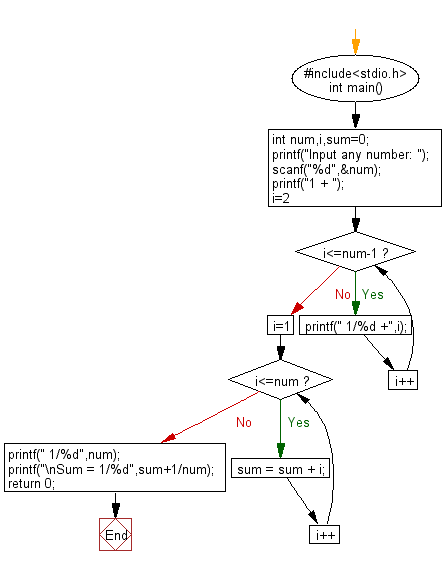﻿ C Program: Display sum of series 1 + 1/2 + 1/3 + ………. + 1/n - w3resource

# C Exercises: Display sum of series 1 + 1/2 + 1/3 + ………. + 1/n

## C Basic Declarations and Expressions: Exercise-59 with Solution

Write a C program to display the sum of series 1 + 1/2 + 1/3 + ………. + 1/n.

Sample Solution:

C Code:

``````#include<stdio.h>
int main() {
int num, i, sum = 0;

// Prompt the user to input a number
printf("Input any number: ");
scanf("%d", &num);

// Display the initial part of the series
printf("1 + ");

// Print the series
for(i = 2; i <= num - 1; i++)
printf(" 1/%d +", i);

// Calculate the sum of the series
for(i = 1; i <= num; i++)
sum = sum + i;

// Display the last term of the series
printf(" 1/%d", num);

// Calculate and display the sum
printf("\nSum = 1/%d", sum + 1/num);

return 0;
}
``````

Sample Output:

```Input any number: 1 +  1/0
Sum = 1/0
```

Flowchart:C programming Code Editor:

What is the difficulty level of this exercise?

Test your Programming skills with w3resource's quiz.

﻿